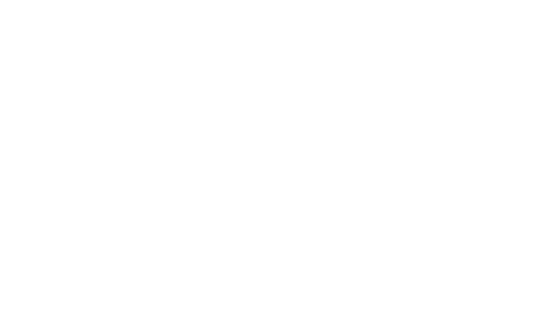# swastik Patterns Programs in C++

swastik Pattern in C++, uses the control statement.

swastik will print according to the input value given by the user, as the program condition is that the user-input should be minimum 4 and maximum 23, so that a better Swastik will be print.

If the user input is less than 4 and greater than 23, then the program will automatically exit.

The goto statement has also been used in the program, here the goto statement will behave like a loop. Which maintains the program execution until the condition is false i.e. the user-input is less than 4 or greater than 23.

That is to say, when the user enters input, the user-input will be checked in the if statement before the swastik is printed whether the input is less than 4 or more than 23.

such as,

``````if(no>23 || no<3) // check user input then execute program
exit(0); // if condition true body of if will execute``````

In the last-end statement of the program, execution from goto statement (goto start;) has been transferred to the program header. Which means the program will execute again, then the user will input, then the condition will be checked in the if statement, then swastik will print, then goto start will transfer to the execution header. This process will continue until the condition in the if statement becomes false.

In the program, different parts of the pattern have been given with comments, along with their definition, which shows which part of the code will print the swastik in the program and also the video output of the program has been given.

create swastik pattern in C++ using control- statement

``````#include<iostream>
#include<stdlib.h>
using namespace std;
int main()
{
int c,r,no;
start:
{         // goto statement label start

cout<<"Enter Number(Minimum 4 and Maximum 23): ";
cin>>no;

if(no%2==0)
c=no+1;
else
c=no;

if(no>23 || no<3)  // check user input then execute program
exit(0);  // if condition true body of if will execute

for(int i=0; i<c; i++)
{
r=c;
for(int j=0; j<r; j++)
{
int s=r-1,m=r/2-1;
if(i==0)
{
//print first row
int x=r/2;
if(j<=x)
cout<<"#";
if(j>=x && j!=s && j!=r/2)
cout<<"8";   //print space into first row
if(j==s)
cout<<"#";
//#######     #

}

if(i!=0 && i<r/2 )
{
//upper part of pattern
if(j==s/2)
cout<<"#";   //print center horizontal line
if(j==s)
cout<<"#";    //print right side horizontal line
if(j!=s/2 && j!=s)
cout<<" ";   // print space
//    #     #
//    #     #
//    #     #
//    #     #
//    #     #
}

//print vertical center line
if(i==c/2)
cout<<"2";
//#############

if(i>r/2 && i<s)
{
//print lower part of pattern
if(j==0)
cout<<"#";  //print first horizontal line
if(j==(r/2+1))
cout<<"#"; // print center horizontal line
if(j!=0 && j!=m)
cout<<" ";
//#     #
//#     #
//#     #
//#     #
//#     #
}

if(i==s)
{
// bottom row of pattern
if(j==0)
cout<<"#";  // print first
if(j>m)
cout<<"#";
if(j!=0 && j<=m)
cout<<" ";  // print space
// #    #######
}
}
cout<<"\n";
}
return 0;
}
goto start;
}``````

OUTPUT

``````Enter Number(Minimum 4 and Maximum 23):-12
#######     #
#     #
#     #
#     #
#     #
#     #
#############
#     #
#     #
#     #
#     #
#     #
#     #######

Enter Number(Minimum 4 and Maximum 23):-``````